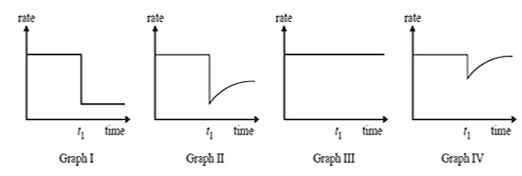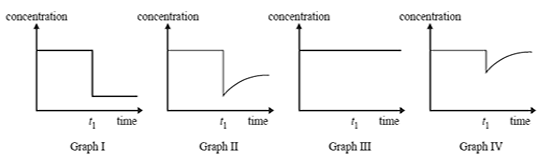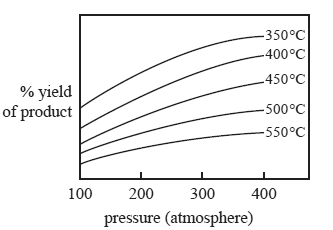Equilibrium 2010 1) The most common method for the industrial production of hydrogen is the steam reforming process, which requires high temperature, high pressure and a Ni catalyst. The equation for this reaction is CH4(g) + H2O(g) => CO(g) + 3H2(g) ΔH = +207 kJ mol–1 i. Write an equilibrium expression for the steam reforming reaction. Solution ii. Le Chatelier's principle indicates that equilibrium yield for the reaction above is favoured by low pressure. Suggest one reason why high pressure is used in the industrial process described above. Solution At 1500 °C the concentrations of the gases in a particular equilibrium mixture were found to be [CH4] = 0.400 M, [CO] = 0.300 M, [H2O] = 0.068 M K = 5.67 M2 at 1500 °C for the reaction. iii. Calculate the molar concentration of H2 in the equilibrium mixture. Solution2) Reactants A and B are placed in a sealed container with a suitable catalyst where they react according to the equation A(g) + B(g) <=> C(g) After the reaction reaches equilibrium, a small amount of a compound is added to the container at time t1. The compound 'poisons' the catalyst and stops it working.Which one of the graphs best represents the rate of the forward reaction versus time? A. Graph I B. Graph II C. Graph III D. Graph IV Solution4) Which one of the graphs best represents the concentration of product C versus time? A. Graph I B. Graph II C. Graph III D. Graph IV Solution5) Nitrosyl chloride (NOCl) is a highly toxic gas that decomposes according to the equation 2NOCl(g) <=>2NO(g) + Cl2(g) To investigate the reaction, 1.2 mol of NOCl(g) is placed in an empty 1.0 L flask and allowed to reach equilibrium. The flask and its contents are kept at a constant temperature. If [Cl2] = 0.20 M at equilibrium, what is the equilibrium concentration of NOCl(g)? A. 0.80 M B. 1.00 M C. 1.10 M D. 1.40 M Solution 6) The following reaction systems are at equilibrium in separate sealed containers. The volumes of the containers are halved at constant temperature. Which reaction has the largest percentage change in the concentration fraction (reaction quotient) immediately after the volume change? A. N2O4(g) <=>2NO2(g) B. H2(g) + I2(g) <=> 2HI(g) C. 2CO2(g) <=> 2CO(g) + O2(g) D. CO(g) + 2H2(g) <=> CH3OH(g) SolutionThe graph below refers to the following gaseous reaction. aA(g) + bB(g) <=> cC(g) + dD(g) The effect of increasing pressure and temperature on the equilibrium yield of the products is shown in the graph below.. 7) Which one of the following statements about the relative number of reactant and product molecules in the balanced equation is true? A. The number of reactant molecules is equal to the number of product molecules. B. The number of reactant molecules is greater than the number of product molecules. C. The number of reactant molecules is less than the number of product molecules. D. The relative number of reactant and product molecules cannot be determined from the data provided. Solution 8) Which one of the following statements about this gaseous reaction is true? A. The reaction is exothermic because the yield increases as the temperature increases. B. The reaction is endothermic because the yield increases as the temperature increases. C. The reaction is exothermic because the yield decreases as the temperature increases. D. The reaction is endothermic because the yield decreases as the temperature increases. Solution